This example shows how to simulate received signal of a monostatic pulse radar to estimate the target range. A monostatic radar has the transmitter collocated with the receiver. The transmitter generates a pulse which hits the target and produces an echo received by the receiver. By measuring the location of the echoes in time, we can estimate the range of a target.

This example focuses on a pulse radar system design which can achieve a set of design specifications. It outlines the steps to translate design specifications, such as the probability of detection and the range resolution, into radar system parameters, such as the transmit power and the pulse width. It also models the environment and targets to synthesize the received signal. Finally, signal processing techniques are applied to the received signal to detect the ranges of the targets.

Design Specifications

The design goal of this pulse radar system is to detect non-fluctuating targets with at least one square meter radar cross section (RCS) at a distance up to 5000 meters from the radar with a range resolution of 50 meters. The desired performance index is a probability of detection (Pd) of 0.9 and probability of false alarm (Pfa) below 1e-6. Since coherent detection requires phase information and, therefore is more computationally expensive, we adopt a noncoherent detection scheme. In addition, this example assumes a free space environment.

pd = 0.9;            % Probability of detection
pfa = 1e-6;          % Probability of false alarm
max_range = 5000;    % Maximum unambiguous range
range_res = 50;      % Required range resolution
tgt_rcs = 1;         % Required target radar cross section

We need to define several characteristics of the radar system such as the waveform, the receiver, the transmitter, and the antenna used to radiate and collect the signal.

Waveform

We choose a rectangular waveform in this example. The desired range resolution determines the bandwidth of the waveform, which, in the case of a rectangular waveform, determines the pulse width.

Another important parameter of a pulse waveform is the pulse repetition frequency (PRF). The PRF is determined by the maximum unambiguous range.

prop_speed = physconst('LightSpeed');   % Propagation speed
pulse_bw = prop_speed/(2*range_res);    % Pulse bandwidth
pulse_width = 1/pulse_bw;               % Pulse width
prf = prop_speed/(2*max_range);         % Pulse repetition frequency
fs = 2*pulse_bw;                        % Sampling rate
waveform = phased.RectangularWaveform(...
'PulseWidth',1/pulse_bw,...
'PRF',prf,...
'SampleRate',fs);

Note that we set the sampling rate as twice the bandwidth.

We assume that the only noise present at the receiver is the thermal noise, so there is no clutter involved in this simulation. The power of the thermal noise is related to the receiver bandwidth. The receiver's noise bandwidth is set to be the same as the bandwidth of the waveform. This is often the case in real systems. We also assume that the receiver has a 20 dB gain and a 0 dB noise figure.

noise_bw = pulse_bw;

'Gain',20,...
'NoiseFigure',0,...
'SampleRate',fs,...
'EnableInputPort',true);

Note that because we are modeling a monostatic radar, the receiver cannot be turned on until the transmitter is off. Therefore, we set the EnableInputPort property to true so that a synchronization signal can be passed from the transmitter to the receiver.

Transmitter

The most critical parameter of a transmitter is the peak transmit power. The required peak power is related to many factors including the maximum unambiguous range, the required SNR at the receiver, and the pulse width of the waveform. Among these factors, the required SNR at the receiver is determined by the design goal of Pd and Pfa, as well as the detection scheme implemented at the receiver.

The relation between Pd, Pfa and SNR can be best represented by a receiver operating characteristics (ROC) curve. We can generate the curve where Pd is a function of Pfa for varying SNRs using the following command:

snr_db = [-inf, 0, 3, 10, 13];
rocsnr(snr_db,'SignalType','NonfluctuatingNoncoherent');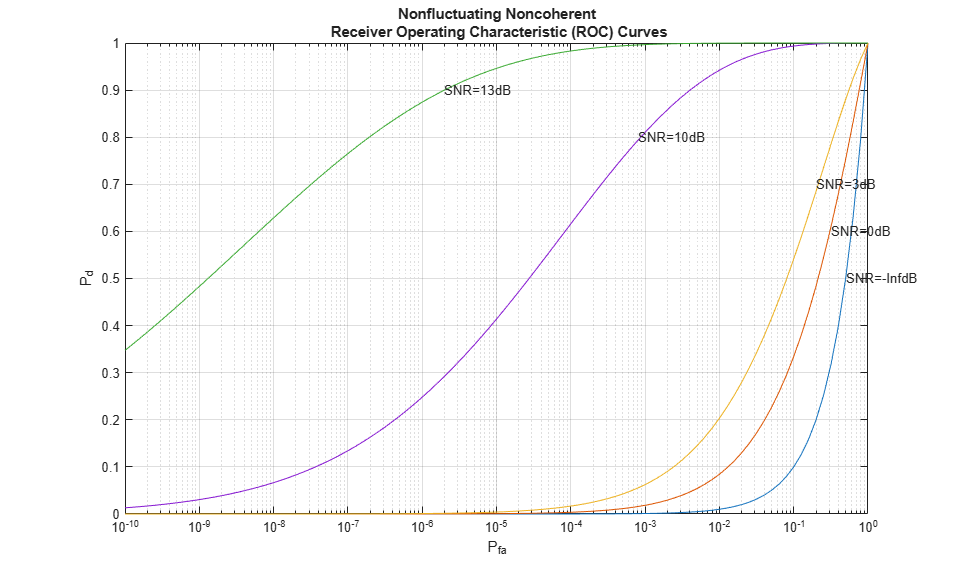The ROC curves show that to satisfy the design goals of Pfa = 1e-6 and Pd = 0.9, the received signal's SNR must exceed 13 dB. This is a fairly high requirement and is not very practical. To make the radar system more feasible, we can use a pulse integration technique to reduce the required SNR. If we choose to integrate 10 pulses, the curve can be generated as

num_pulse_int = 10;
rocsnr([0 3 5],'SignalType','NonfluctuatingNoncoherent',...
'NumPulses',num_pulse_int);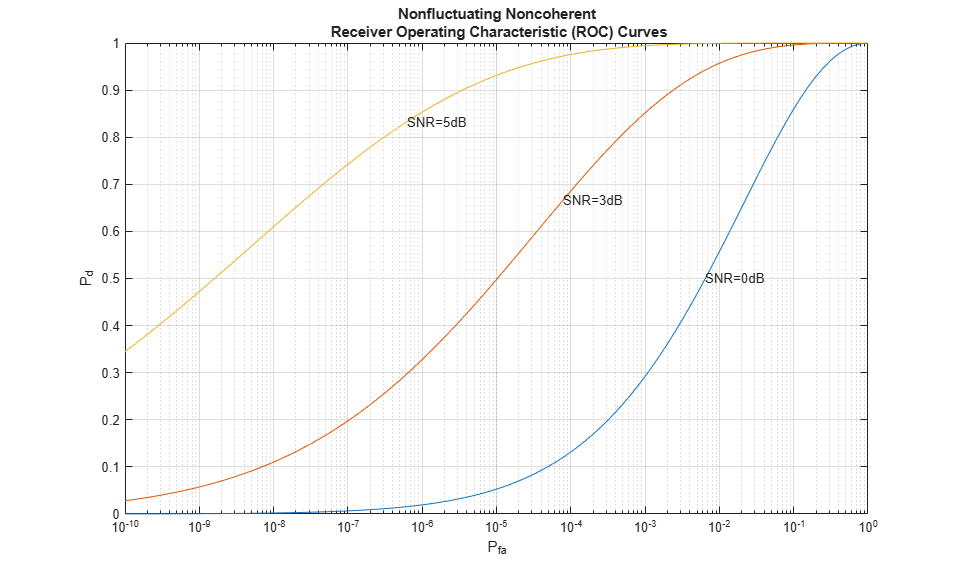We can see that the required power has dropped to around 5 dB. Further reduction of SNR can be achieved by integrating more pulses, but the number of pulses available for integration is normally limited due to the motion of the target or the heterogeneity of the environment.

The approach above reads out the SNR value from the curve, but it is often desirable to calculate only the required value. For the noncoherent detection scheme, the calculation of the required SNR is, in theory, quite complex. Fortunately, there are good approximations available, such as Albersheim's equation. Using Albersheim's equation, the required SNR can be derived as

snr_min = albersheim(pd, pfa, num_pulse_int)
snr_min =

4.9904

Once we obtain the required SNR at the receiver, the peak power at the transmitter can be calculated using the radar equation. Here we assume that the transmitter has a gain of 20 dB.

To calculate the peak power using the radar equation, we also need to know the wavelength of the propagating signal, which is related to the operating frequency of the system. Here we set the operating frequency to 10 GHz.

tx_gain = 20;

fc = 10e9;
lambda = prop_speed/fc;

peak_power = ((4*pi)^3*noisepow(1/pulse_width)*max_range^4*...
db2pow(snr_min))/(db2pow(2*tx_gain)*tgt_rcs*lambda^2)
peak_power =

5.2265e+03

Note that the resulting power is about 5 kW, which is very reasonable. In comparison, if we had not used the pulse integration technique, the resulting peak power would have been 33 kW, which is huge.

With all this information, we can configure the transmitter.

transmitter = phased.Transmitter(...
'Gain',tx_gain,...
'PeakPower',peak_power,...
'InUseOutputPort',true);

Again, since this example models a monostatic radar system, the InUseOutputPort is set to true to output the status of the transmitter. This status signal can then be used to enable the receiver.

In a radar system, the signal propagates in the form of an electromagnetic wave. Therefore, the signal needs to be radiated and collected by the antenna used in the radar system. This is where the radiator and the collector come into the picture.

In a monostatic radar system, the radiator and the collector share the same antenna, so we will first define the antenna. To simplify the design, we choose an isotropic antenna. Note that the antenna needs to be able to work at the operating frequency of the system (10 GHz), so we set the antenna's frequency range to 5-15 GHz.

We assume that the antenna is stationary.

antenna = phased.IsotropicAntennaElement(...
'FrequencyRange',[5e9 15e9]);

sensormotion = phased.Platform(...
'InitialPosition',[0; 0; 0],...
'Velocity',[0; 0; 0]);

With the antenna and the operating frequency, we define both the radiator and the collector.

'Sensor',antenna,...
'OperatingFrequency',fc);

collector = phased.Collector(...
'Sensor',antenna,...
'OperatingFrequency',fc);

This completes the configuration of the radar system. In the following sections, we will define other entities, such as the target and the environment that are needed for the simulation. We will then simulate the signal return and perform range detection on the simulated signal.

System Simulation

Targets

To test our radar's ability to detect targets, we must define the targets first. Let us assume that there are 3 stationary, non-fluctuating targets in space. Their positions and radar cross sections are given below.

tgtpos = [[2024.66;0;0],[3518.63;0;0],[3845.04;0;0]];
tgtvel = [[0;0;0],[0;0;0],[0;0;0]];
tgtmotion = phased.Platform('InitialPosition',tgtpos,'Velocity',tgtvel);

tgtrcs = [1.6 2.2 1.05];

Propagation Environment

To simulate the signal, we also need to define the propagation channel between the radar system and each target.

channel = phased.FreeSpace(...
'SampleRate',fs,...
'TwoWayPropagation',true,...
'OperatingFrequency',fc);

Because this example uses a monostatic radar system, the channels are set to simulate two way propagation delays.

Signal Synthesis

We are now ready to simulate the entire system.

The synthesized signal is a data matrix with the fast time (time within each pulse) along each column and the slow time (time between pulses) along each row. To visualize the signal, it is helpful to define both the fast time grid and slow time grid.

fast_time_grid = unigrid(0,1/fs,1/prf,'[)');
slow_time_grid = (0:num_pulse_int-1)/prf;

The following loop simulates 10 pulses of the receive signal.

We set the seed for the noise generation in the receiver so that we can reproduce the same results.

% Pre-allocate array for improved processing speed
rxpulses = zeros(numel(fast_time_grid),num_pulse_int);

for m = 1:num_pulse_int

% Update sensor and target positions
[sensorpos,sensorvel] = sensormotion(1/prf);
[tgtpos,tgtvel] = tgtmotion(1/prf);

% Calculate the target angles as seen by the sensor
[tgtrng,tgtang] = rangeangle(tgtpos,sensorpos);

% Simulate propagation of pulse in direction of targets
pulse = waveform();
[txsig,txstatus] = transmitter(pulse);
txsig = channel(txsig,sensorpos,tgtpos,sensorvel,tgtvel);

% Reflect pulse off of targets
tgtsig = target(txsig);

% Receive target returns at sensor
rxsig = collector(tgtsig,tgtang);
end

Range Detection

Detection Threshold

The detector compares the signal power to a given threshold. In radar applications, the threshold is often chosen so that the Pfa is below a certain level. In this case, we assume the noise is white Gaussian and the detection is noncoherent. Since we are also using 10 pulses to do the pulse integration, the signal power threshold is given by

threshold = npower * db2pow(npwgnthresh(pfa,num_pulse_int,'noncoherent'));

We plot the first two received pulses with the threshold

num_pulse_plot = 2;
fast_time_grid,slow_time_grid,num_pulse_plot);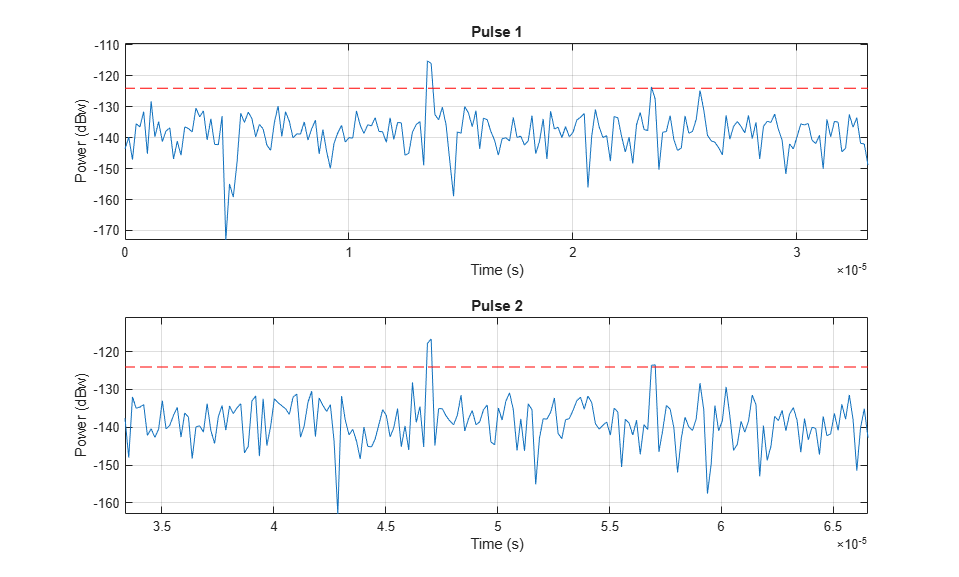The threshold in these figures is for display purpose only. Note that the second and third target returns are much weaker than the first return because they are farther away from the radar. Therefore, the received signal power is range dependent and the threshold is unfair to targets located at different ranges.

Matched Filter

The matched filter offers a processing gain which improves the detection threshold. It convolves the received signal with a local, time-reversed, and conjugated copy of transmitted waveform. Therefore, we must specify the transmitted waveform when creating our matched filter. The received pulses are first passed through a matched filter to improve the SNR before doing pulse integration, threshold detection, etc.

matchingcoeff = getMatchedFilter(waveform);
matchedfilter = phased.MatchedFilter(...
'Coefficients',matchingcoeff,...
'GainOutputPort',true);
[rxpulses, mfgain] = matchedfilter(rxpulses);

The matched filter introduces an intrinsic filter delay so that the locations of the peak (the maximum SNR output sample) are no longer aligned with the true target locations. To compensate for this delay, in this example, we will move the output of the matched filter forward and pad the zeros at the end. Note that in real systems, because the data is collected continuously, there is really no end of it.

matchingdelay = size(matchingcoeff,1)-1;
rxpulses = buffer(rxpulses(matchingdelay+1:end),size(rxpulses,1));

The threshold is then increased by the matched filter processing gain.

threshold = threshold * db2pow(mfgain);

The following plot shows the same two pulses after they pass through the matched filter.

fast_time_grid,slow_time_grid,num_pulse_plot);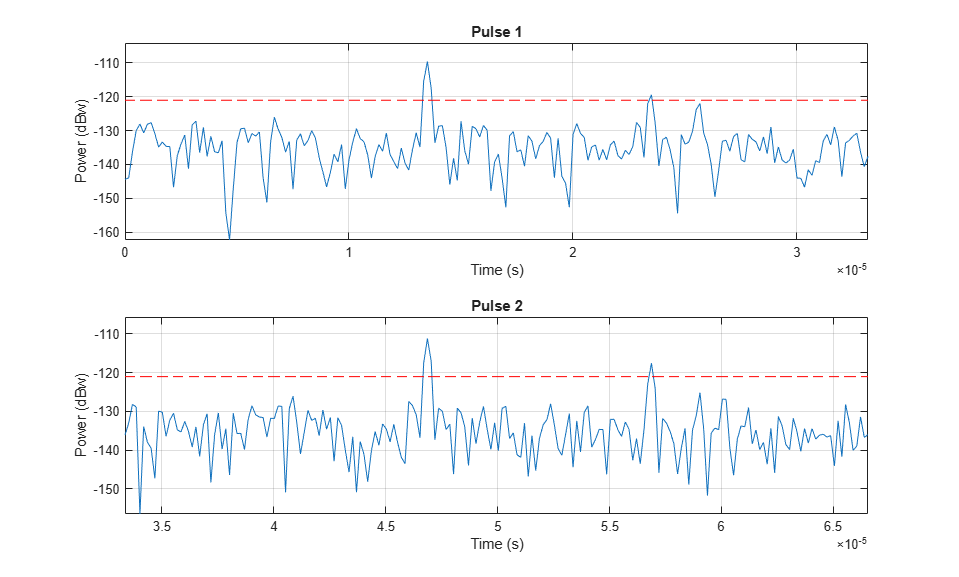After the matched filter stage, the SNR is improved. However, because the received signal power is dependent on the range, the return of a close target is still much stronger than the return of a target farther away. Therefore, as the above figure shows, the noise from a close range bin also has a significant chance of surpassing the threshold and shadowing a target farther away. To ensure the threshold is fair to all the targets within the detectable range, we can use a time varying gain to compensate for the range dependent loss in the received echo.

To compensate for the range dependent loss, we first calculate the range gates corresponding to each signal sample and then calculate the free space path loss corresponding to each range gate. Once that information is obtained, we apply a time varying gain to the received pulse so that the returns are as if from the same reference range (the maximum detectable range).

range_gates = prop_speed*fast_time_grid/2;

tvg = phased.TimeVaryingGain(...
'RangeLoss',2*fspl(range_gates,lambda),...
'ReferenceLoss',2*fspl(max_range,lambda));

rxpulses = tvg(rxpulses);

Now let's plot the same two pulses after the range normalization

fast_time_grid,slow_time_grid,num_pulse_plot);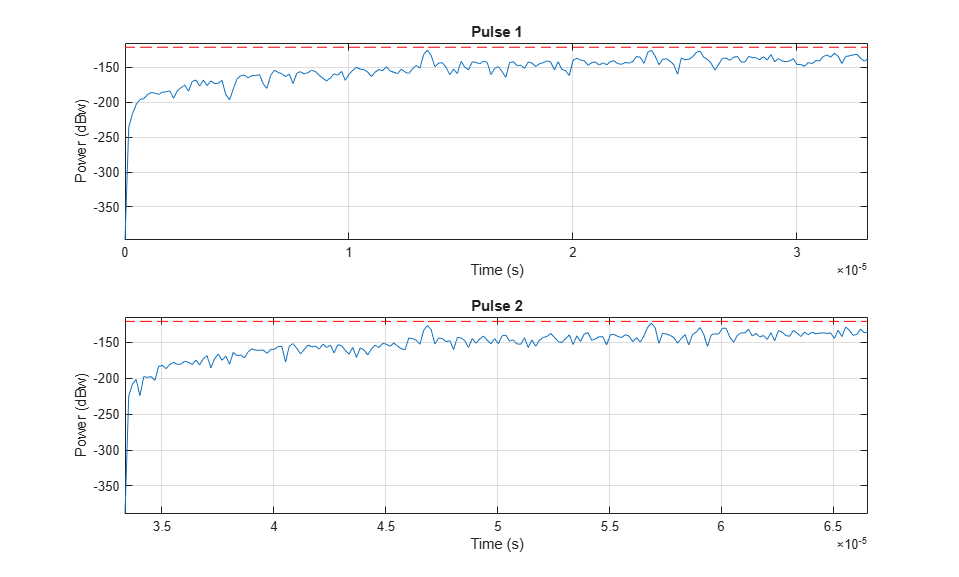The time varying gain operation results in a ramp in the noise floor. However, the target return is now range independent. A constant threshold can now be used for detection across the entire detectable range.

Notice that at this stage, the threshold is above the maximum power level contained in each pulse. Therefore, nothing can be detected at this stage yet. We need to perform pulse integration to ensure the power of returned echoes from the targets can surpass the threshold while leaving the noise floor below the bar. This is expected since it is the pulse integration which allows us to use the lower power pulse train.

Noncoherent Integration

We can further improve the SNR by noncoherently integrating (video integration) the received pulses.

rxpulses = pulsint(rxpulses,'noncoherent');

fast_time_grid,slow_time_grid,1);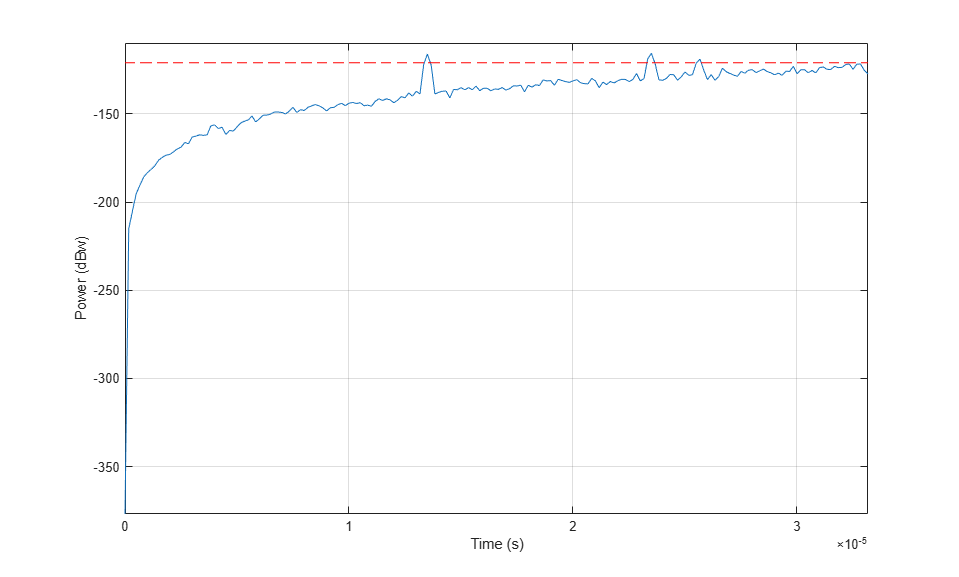After the video integration stage, the data is ready for the final detection stage. It can be seen from the figure that all three echoes from the targets are above the threshold, and therefore can be detected.

Range Detection

Finally, the threshold detection is performed on the integrated pulses. The detection scheme identifies the peaks and then translates their positions into the ranges of the targets.

[~,range_detect] = findpeaks(rxpulses,'MinPeakHeight',sqrt(threshold));

The true ranges and the detected ranges of the targets are shown below:

true_range = round(tgtrng)
range_estimates = round(range_gates(range_detect))
true_range =

2025        3519        3845

range_estimates =

2025        3525        3850

Note that these range estimates are only accurate up to the range resolution (50 m) that can be achieved by the radar system.

Summary

In this example, we designed a radar system based on a set of given performance goals. From these performance goals, many design parameters of the radar system were calculated. The example also showed how to use the designed radar to perform a range detection task. In this example, the radar used a rectangular waveform. Interested readers can refer to Waveform Design to Improve Range Performance of an Existing System for an example using a chirp waveform.